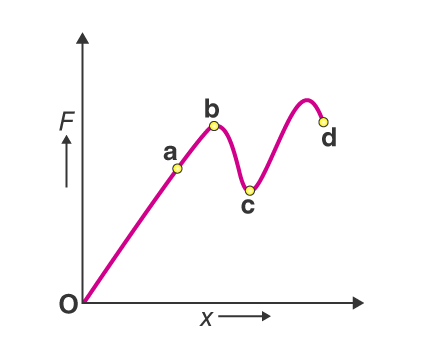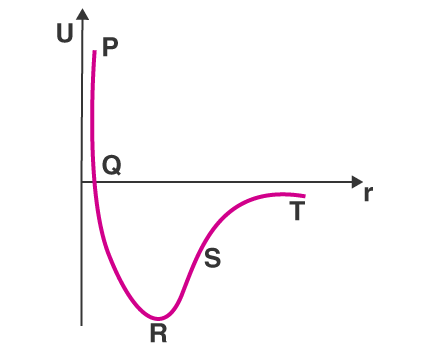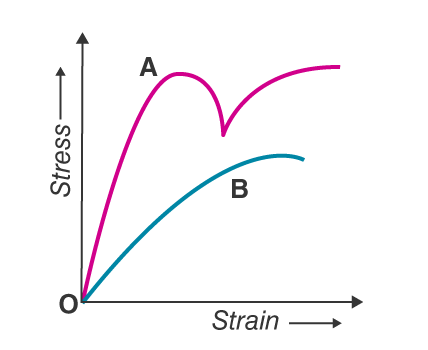# Properties of Bulk Matter MCQs for NEET

Properties of bulk matter include key concepts such as stress, strain, elasticity, Hook’s law, and other concepts related to fluids such as Pascal’s law, viscosity, surface energy, etc.

Stress is the internal restoring force acting on a deformed body per unit area. While the strain is the ratio of change in the shape of the body to the original shape of the body. According to Hook’s law stress is directly proportional to the strain within the elastic limits.

Modulus of elasticity for a body is defined as the ratio of stress to its corresponding strain within the elastic limit. There are three types of modulus of elasticity and they are:

• Young’s modulus of elasticity, Y: It is defined as the ratio of normal stress to the longitudinal strain within the elastic limit.
• Bulk modulus of elasticity, K: It is defined as the ratio of normal stress to the volumetric strain within the elastic limit.
• Modulus of rigidity, η: It is the ratio of tangential stress to shearing strain
1. Hook’s law is used for defining which of the following:(a) Elastic limit(b) Stress(c) Strain(d) Modulus of elasticity

2. What happens to the modulus of elasticity if the temperature increases?(a) Remains constant(b) Increases(c) Decreases(d) Becomes zero

3. What is the modulus of rigidity of a liquid(a) Zero(b) Non-zero constant(c) Infinite(d) There is no modulus of rigidity

4. When an iron needle is slowly placed on the surface of the water, it floats because(a) It is dependent on the shape(b) Surface tension is responsible(c) The density of the material used in the needle is lesser than that of water(d) Water is displaced which is equal to the weight of the needle

Answer: (b) Surface tension is responsible

5. Which of the following is responsible for the change in the shape of a regular body(a) Metallic strain(b) Longitudinal strain(c) Shearing strain(d) Volume stress

6. 1:2 is the ratio of the lengths of the two wires A and B which are made from the same material. 2:1 is the ratio of their diameter. What will be the ratio of their length if the wires are stretched by the same force?(a) 2:1(b) 1:8(c) 1:4(d) 8:1

7. Following is the graph between applied force, F and strain, x for a thin uniform wire. At which point does the wire behave like a liquid in the graph?(a) oa(b) cd(c) bc

(d) ab

8. Following is the graph between potential energy, U and the distance, r for diatomic molecules. Which of the following are the points for maximum and minimum attraction(a) S and T(b) T and S(c) R and S

(d) R and T

9. Stress versus strain curve for two materials A and B are given below. What is inferred from the graph(a) Material A is brittle and material B is ductile(b) Both the materials are ductile(c) Material A is ductile and material B is brittle

(d) Both the materials are brittle

Answer: (c) Material A is ductile and material B is brittle

10. What is the fractional decrease in the radius of the spherical object that has a bulk modulus B and is subjected to uniform pressure p(a) $$\frac{B}{3p}$$(b) $$\frac{p}{B}$$(c) $$\frac{p}{3B}$$(d) $$\frac{3p}{B}$$

Answer: (c) $$\frac{p}{3B}$$

11. How will the volume of elasticity change if Young’s modulus of the material is equal to 3 times the modulus of rigidity(a) Zero(b) 2 x 1010 N/m2(c) 3 x 1010 N/m2(d) Infinity

12. What is the relationship between modulus of rigidity η, bulk modulus K, and Young’s modulus Y?(a) $$Y=\frac{9\eta K}{3+K}$$(b) $$Y=\frac{3\eta K}{9\eta+K}$$(c) $$\frac{9YK}{Y+3K}$$(d) $$Y=\frac{9\eta K}{\eta +3K}$$

Answer: (d) $$Y=\frac{9\eta K}{\eta +3K}$$